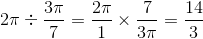# SAT II Math II : Graphing Parametric Functions

## Example Questions

### Example Question #1 : Graphing Parametric Functions

Give the period of the graph of the equation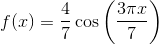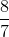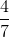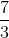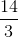The period of the graph of a cosine function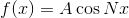is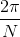, or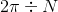Since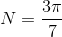, the period is